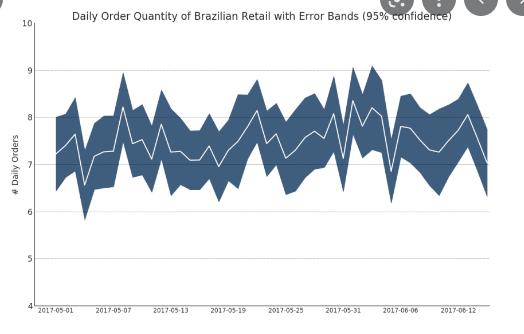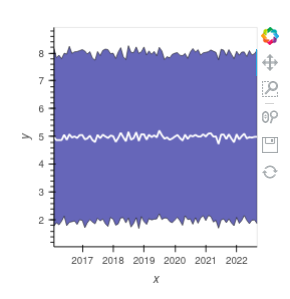# 5 year range ; color area inbetween - might be simple?

hey guys I have a dataframe like this ; the 5 yr avg is only on 2nd row bcuz of space ; but its all in 1 dataframe… horizontally…

I would like to create something like this:
where I have the 5 yr max at the top, 5 year min at bottom ; area is shaded… and then have the 5 year average line… I also want the current year and the previous year lines … soo all on the same plot… I’ve seen super long matplotlib examples and was wondering if hvplot has some magic or more concise code I could look at / learn from / get help with . Thank you .You can’t create the plot with the data you have. The plot you have shown is properly created from the statistical values of a time series.

If you have time series data, an example of how it could be done is shown below.

``````import holoviews as hv
import numpy as np
import pandas as pd

hv.extension("bokeh")

# Creating time series data
dates = pd.date_range("2016-01-01", "2022-08-22", freq="H")
data = np.random.normal(5, 3, dates.size)
df = pd.DataFrame(data=data, columns=["data"], index=dates)

# Getting statistical values of data
df_stat = df.resample("M").mean().rename({"data": "mean"}, axis=1)
df_stat["std"] = df.resample("M").std().rename({"data": "std"}, axis=1)
df_stat

# creating the plot
error = hv.Spread((df_stat.index, df_stat["mean"], df_stat["std"])).opts(
color="darkblue"
)
curve = hv.Curve((df_stat.index, df_stat["mean"])).opts(color="white")

error * curve
``````2 Likes

wicked, thanks Hoxbro !

1 Like